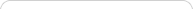##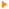Teaching with Sketchpad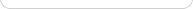•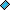- plays a video
•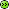- opens a web page# Algebra

NOTE: If you are using Internet Explorer and are unable to download the .zip activity files, right-click the download link and choose Save Target As to save to your computer.

##Tiling in a Frame: Multiplying Polynomials

Students use Sketchpad algebra tiles to multiply polynomials. Using the polynomial factors as dimensions, they build rectangles out of tiles. The area of the completed rectangle represents the product.

##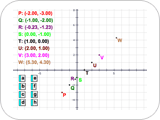Points Lining Up in the Plane

Students are informally, experientially introduced to the relationship between descriptions of coordinate patterns and graphs in the Cartesian plane. Too often students don’t really understand the connection between an equation and its graph. This activity fosters the understanding that graphs depict the set of points whose coordinates satisfy an equation.

##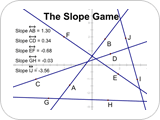The Slope Game

Students acquire an intuitive feel for slope as they construct and play a game in which one player rearranges lines on the screen and the other player tries to match each line with its slope measurement. The game can be modified to play alone as well.

##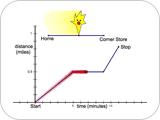Mellow Yellow: Interpreting Graphs

Students interpret linear piecewise time-distance graphs that represent different stories about a character, Mellow Yellow. They decide whether a given graph corresponds to the motions (walking fast, walking slow, stopping, going backward) described in the story. Students then create stories based on given graphs and create graphs based on given stories.

##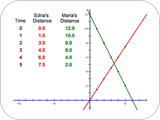Hikers: Solving Through Multiple Representations

Students use tables, graphs, and equations to represent and solve a real-world problem about two hikers walking at different speeds in opposite directions along the same trail.

##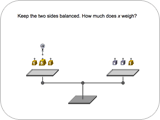Balancing with Balloons: Solving Equations with Negatives

Students use a Sketchpad pan balance model to solve a sequence of equations with positive numbers and variables, represented by weights, and negative numbers and variables, represented by balloons. As the problems increase in difficulty, students transfer the manipulation of the pan balance to solving equations independent of the balance.

##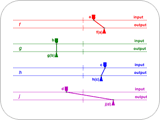Introducing Dynagraphs

Students explore dynagraphs, an alternative to Cartesian graphs, to develop a feel for various types of functional relationships.

##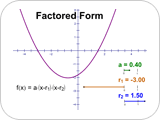Parabolas in Factored Form

Students plot a quadratic function in factored form, investigate the relationship between the equation and its graph, and use their observations to create functions from various descriptions of their graphs.

##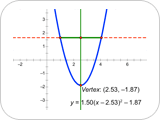Quadratic Quandary: Finding the Vertex of a Parabola

Students convert quadratic functions between standard, vertex, and factored forms. They check their results by changing parameters and comparing the graphs.

##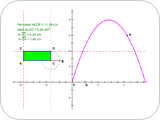A Rectangle with Maximum Area

Students explore areas of rectangles whose perimeter is fixed. They make a conjecture about what type of rectangle has the most area for a given perimeter and check their conjecture by plotting the side length and area of the rectangle on the coordinate plane.